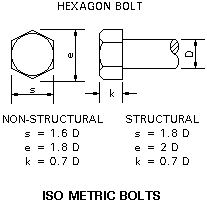# Finding the Force on a bolt from a wrench

## Homework Statement

In an Effort to tighten a bolt, a force F is applied to the end of a wrench that has a length L perpendicular to the wrench. If the bolt itself has a radius of b, how much force is applied to the bolt

## Homework Equations

∑τ=RFsinθ ΣF=ma ∑T=Iα

## The Attempt at a Solution

I tried summing the torques
∑τ=LF+bF=(I_bolt+I_wrench)α

a)F
b)Fb/L
c)FL/b
d)FL/(b+L)
e)bL/F

I'm not even sure how you would solve this to get anything like that or why there is an F in the answer when we're solving for F. My friend says this is a conceptual engineering problems and that c makes sense since the Force is multiplied by the length of the bolt (they are really looking for torque) but divided by the length of the wrench.

BvU
Homework Helper
how much force is applied to the bolt
That's not the title of your thread. You sure they aren't out to trick you ?

And: make a drawing

Last edited:
here's a pic drawn in paint

#### Attachments

•wrench physics.png
967 bytes · Views: 479
BvU
Homework Helper
L is a bit vague, don't you think ?

And do you think people mean that when they mention the radius of a bolt ? In the civilized world the M10 bolt has a radius of 5 mm, but the head is a bit bigger !

2. Homework Equations
∑τ=RFsinθ
ΣF=ma
∑T=Iα

3. The Attempt at a Solution
I tried summing the torques
∑τ=LF+bF=(I_bolt+I_wrench)α

Note that there is only one F in your drawing !

You be the judge: answering the question literally is a). But the force the thread of the bolt exerts on the thread on the inside of the hole (or nut) is c) as your friend sort of indicated. This multiplication factor is the reason wrenches exist(Note that the length of a bolt is in general not the same as the radius...)

You know the torque from L x F. (angle is 90 degrees). At the thread of the bolt the torque is the same !

None of the answers has the dimension of torque ( Newton x meter or better: Force x length). All of them have the dimension of a force.

So are we looking for a ratio of the torques to find the Force?

L is a bit vague, don't you think ?

And do you think people mean that when they mention the radius of a bolt ? In the civilized world the M10 bolt has a radius of 5 mm, but the head is a bit bigger !

Note that there is only one F in your drawing !

You be the judge: answering the question literally is a). But the force the thread of the bolt exerts on the thread on the inside of the hole (or nut) is c) as your friend sort of indicated. This multiplication factor is the reason wrenches exist(Note that the length of a bolt is in general not the same as the radius...)

You know the torque from L x F. (angle is 90 degrees). At the thread of the bolt the torque is the same !

None of the answers has the dimension of torque ( Newton x meter or better: Force x length). All of them have the dimension of a force.

Oh a radius of a bolt is the round thing before the threat, is the force applied there?

#### Attachments

BvU
Homework Helper
No. That's a specific type of bolt and that radius has little to do with the exercise.

I meant the radius of the shaft: so D/2 in the drawing belowSo are we looking for a ratio of the torques to find the Force?
I tried to explain that there is only one torque and that the ratio of the forces at L and at b is the ratio of b and L : ##\tau = L \times F_L ## and ##\tau = b \times F_b##

Last edited:
No. That's a specific type of bolt and that radius has little to do with the exercise.

I meant the radius of the shaft: so D/2 in the drawing belowI tried to explain that there is only one torque and that the ratio of the forces at L and at b is the ratio of L and b : ##\tau = L \times F_L ## and ##\tau = b \times F_b##

Ohhh that makes so much sense thanks